# Examples of joint grid discretization

## Example 1. Nonlinear curves using k-means clustering with a fixed number of clusters

require(GridOnClusters)
x = rnorm(50)
y = sin(x)
z = cos(x)
data = cbind(x, y, z)
res = discretize.jointly(data, k=3) # using a specified k
plotGOCpatterns(data, res)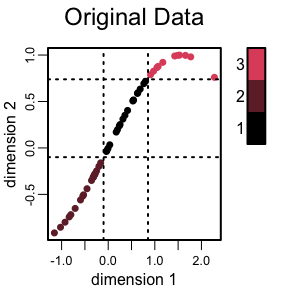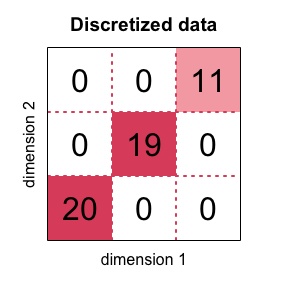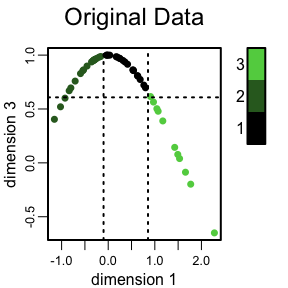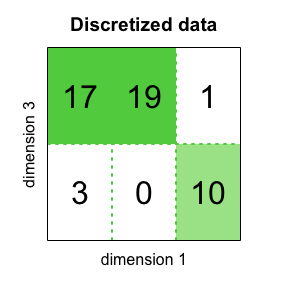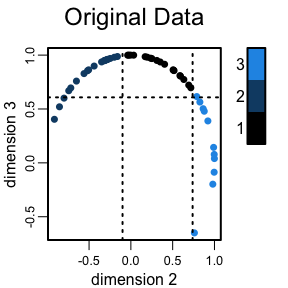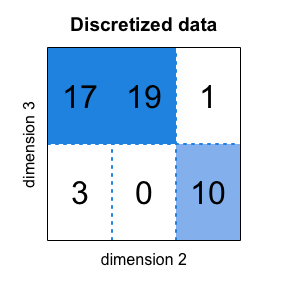Example 1. Nonlinear curves using k-means clustering with a fixed number of clusters.

## Example 2. Nonlinear curves and patterns using k-means clustering with a range for the number of clusters

 x = rnorm(100)
y = log1p(abs(x))
z = ifelse(x >= -0.5 & x <= 0.5, 0, 1) + rnorm(100, 0, 0.1)
data = cbind(x, y, z)
res = discretize.jointly(data, k=c(2:3)) # using a range of k
plotGOCpatterns(data, res)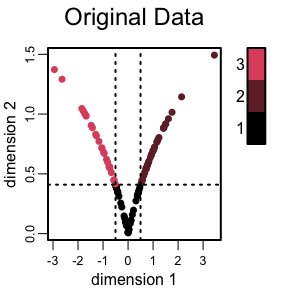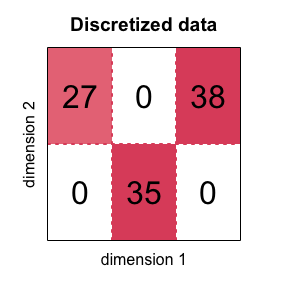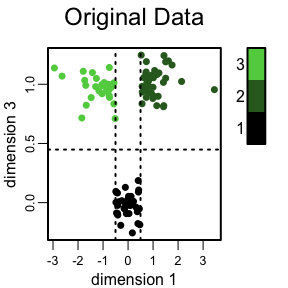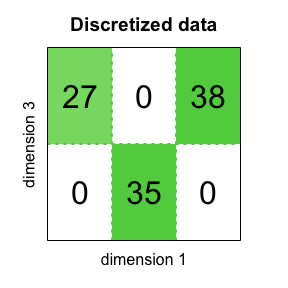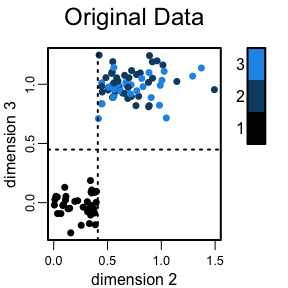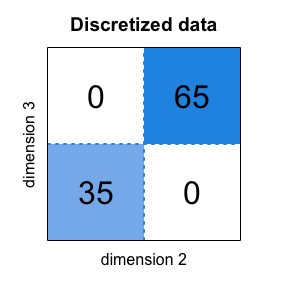Example 2. Using a range for the number of k-means clusters

## Example 3. Using the partition around medoids clustering method

 # using a clustering method other than k-means
x = rnorm(100)
y = log1p(abs(x))
z = sin(x)
data = cbind(x, y, z)

# pre-cluster the data using partition around medoids (PAM)
cluster_label = cluster::pam(x=data, diss = FALSE, metric = "euclidean", k = 4)\$clustering
res = discretize.jointly(data, cluster_label = cluster_label)
plotGOCpatterns(data, res)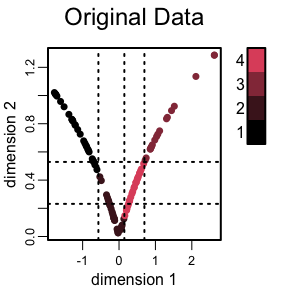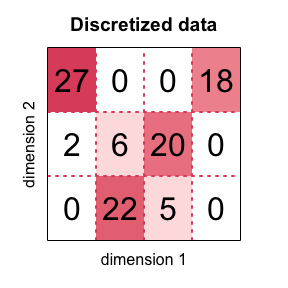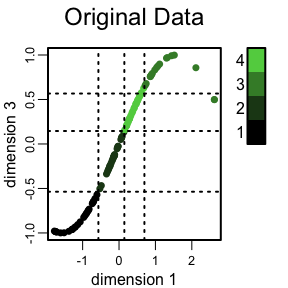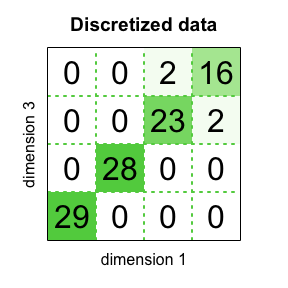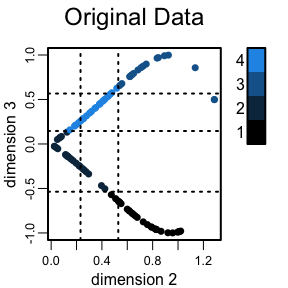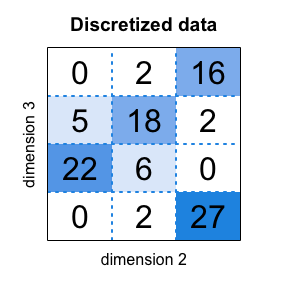Example 3. Using the partition around medoids clustering method.